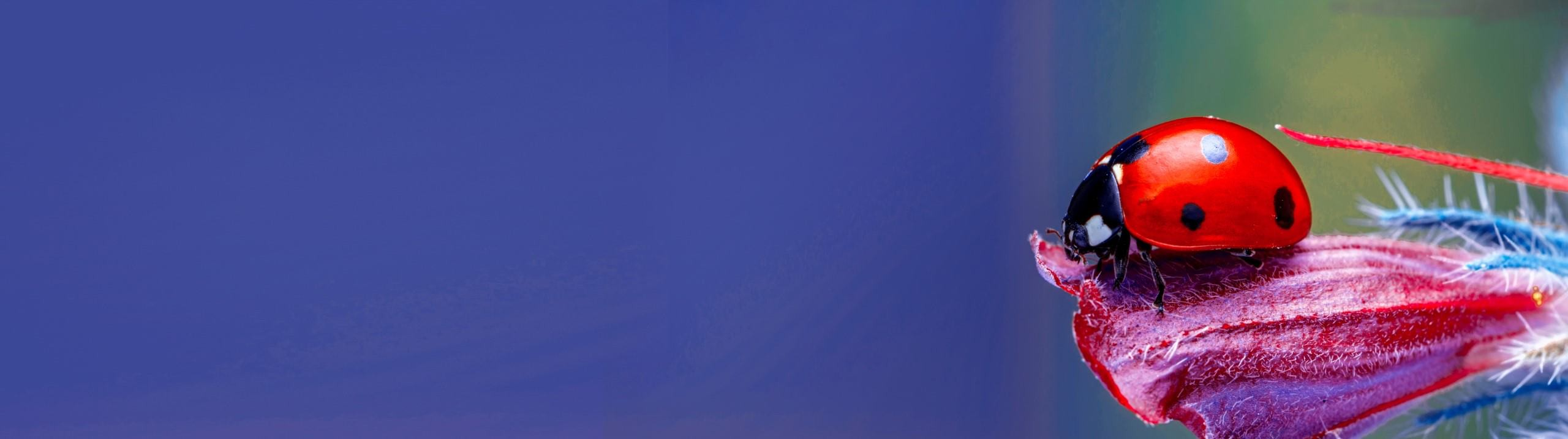## General Biology

Learn the toughest concepts covered in Biology1&2 with step-by-step video tutorials and practice problems by world-class tutors

1. Introduction to Biology

# Scientific Method

1
concept

## Scientific Method6m
Play a video:
2
concept

## Predictions, Hypotheses, & Theories8m
Play a video:
3
example

## Scientific Method Example 11m
Play a video:
all right. So here we have an example problem that says a scientist observed a new phenomenon and wonders how it happens What is the next step in their study? And we've got these five potential answer options down below. And so what we need to realize is that when the scientists observes the new phenomenon and then wonders how it happens, this is really telling us that the first two steps of the scientific method have been completed. And so the observation step and the question step have both been completed already. And so really, what we're looking for here in this, uh, problem is the next step, which would be the third step of the scientific method. And so when we consider that, of course, we know that the third step is going to be to create or formulate the hypothesis on. So that would be to, um, essentially essentially hypothesized on DSO Option E here is going to be the correct answer for the next step. Observe has already happened. Experiment does not take place until after the hypothesis has been generated. So that won't be the next one. Uh, designed the experiment once again. That is not going to happen until after the hypothesis has been created, and then peer review is one of the last steps of the process. And so, once again, option E hypothesize is the correct answer here, and that concludes this example. So I'll see you guys in our next video.
4
Problem

Which of the following shows the best order of steps of the scientific method?

5
Problem

A scientist observes that even after sterilizing a broth, cells reappear in the broth over time. The scientist then asks: “Why do cells reappear in the broth after sterilization?” and designs/conducts an experiment. Considering this, appropriately label each of the following blanks as either a “prediction, hypothesis or theory.”

________________: Cells will only appear in the broth exposed to a source of preexisting cells.

________________: Cells appear only when preexisting cells grow & divide; therefore, cells only appear in exposed broths.

________________: All organisms consist of cells & all cells come from preexisting cells.

6
concept

## Basic Theories of Biology3m
Play a video: